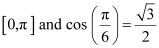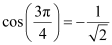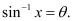Request a Free Counselling Session from our Expert Mentor# NCERT Solution for Class 12 Maths Chapter 2

Get Free NCERT Solutions for Class 12 Mathematics Chapter 2 Inverse Trigonometry, set up after careful examination by profoundly experienced Mathematics instructors, at Swastik Classes. NCERT Solutions are extremely helpful while doing your homework and also for your Class 12 board exam preparation. We have given bit-by-bit answers to every one of the questions given in the NCERT class 12 Mathematics course reading. This solution is free to download and the questions are systematically arranged for your ease of preparation and in solving different types of questions. To score good marks, students are encouraged to get familiar with these NCERT solutions of Chapter 2 Inverse Trigonometry.

## Answers of Mathematics NCERT solutions for class 12 Chapter 2 -Inverse Trigonometric

Question 1:

Find the principal value of

Let sin-1  Then sin

We know that the range of the principal value branch of sin−1 is

and

Therefore, the principal value of is

Question 2:

Find the principal value of

We know that the range of the principal value branch of cos−1 is.

Therefore, the principal value of is

Question 3:

Find the principal value of cosec−1 (2)

Let cosec−1 (2) = y. Then,

We know that the range of the principal value branch of cosec−1 is

Therefore, the principal value of  is

Question 4:

Find the principal value of

We know that the range of the principal value branch of tan−1 is

Therefore, the principal value of  is

Question 5:

Find the principal value of

We know that the range of the principal value branch of cos−1 is

Therefore, the principal value of  is

Question 6:

Find the principal value of tan−1 (−1)

Let tan−1 (−1) = y. Then,

We know that the range of the principal value branch of tan−1 is

Therefore, the principal value of  is

Question 7:

Find the principal value of

We know that the range of the principal value branch of sec−1 is

Therefore, the principal value of  is

Question 8:

Find the principal value of

We know that the range of the principal value branch of cot−1 is (0,π) and

Therefore, the principal value of is

Question 9:

Find the principal value of

We know that the range of the principal value branch of cos−1 is [0,π] and.

Therefore, the principal value of is

Question 10:

Find the principal value of

We know that the range of the principal value branch of cosec−1 is

Therefore, the principal value of  is

Question 11:

Find the value of

Question 12:

Find the value of

Question 13:

Find the value of if sin−1 y, then

(A)   (B)  (C)  (D)

It is given that sin−1 y.

We know that the range of the principal value branch of sin−1 is

Therefore,.

Question 14:

Find the value of is equal to

(A)  (B)   (C)   (D)

### Exercise 1.2

Question 1:

Prove

To prove:

Let x = sinθ. Then,We have,

R.H.S.

= 3θ

= L.H.S.

Question 2:

Prove

To prove:

Let x = cosθ. Then, cos−1 x =θ.

We have,

Question 3:

Prove

To prove:

Question 4:

Prove

To prove:

Question 5:

Write the function in the simplest form:

Question 6:

Write the function in the simplest form:

Put x = cosec θ ⇒ θ = cosec−1 x

Question 7:

Write the function in the simplest form:

Question 8:

Write the function in the simplest form:

tan-1cosx-sinxcosx+sinx=tan-11-sinxcosx1+sinxcosx=tan-11-tanx1+tanx

y=tan-11-tanx1+tanx

tany=1-tanx1+tanx

tany=(tan4-tanx1+tan4tanx)

tany=ta4-x

y=4-x

Question 9:

Write the function in the simplest form:

Question 10:

Write the function in the simplest form:

Question 11:

Find the value of

Let Then,

Question 12:

Find the value of

Question 13:

Find the value of

Let x = tan θ. Then, θ = tan−1 x.

Let y = tan Φ. Then, Φ = tan−1 y.

Question 14:

If then find the value of x.

On squaring both sides, we get:

Hence, the value of x is

Question 15:

If then find the value of x.

Hence, the value of x is

Question 16:

Find the values of

We know that sin−1 (sin x) = x if which is the principal value branch of sin−1x.

Here,

Now, can be written as:

Question 17:

Find the values of

We know that tan−1 (tan x) = x ifwhich is the principal value branch of tan−1x.

Here,.

Now,

Question 18:

Find the values of

Let Then,

Question 19:

Find the values of is equal to

(A)   (B)   (C)   (D)

We know that cos−1 (cos x) = x if which is the principal value branch of cos−1x.

Here,

Now, can be written as:

cos 7π6 =cos π+6 =-cos 6 =cos5π6 = 5π6

θ = – cos θ

Question 20:

Find the values of is equal to

(A)   (B)   (C)   (D) 1

Let Then,

We know that the range of the principal value branch of is .

Question 21:

Find the values of is equal to

(A)   (B)   (C) 0  (D)

Let Then, where

We know that the range of the principal value branch of is

Let

The range of the principal value branch of is

### Miscellaneous Exercise

Question 1:

Find the value of

We know that cos−1 (cos x) = x if which is the principal value branch of cos −1x.

Here,

Now, can be written as:

Question 2:

Find the value of

We know that tan−1 (tan x) = x if which is the principal value branch of tan −1x.

Here,

Now,

can be written as:

Question 3:

Prove

Now, we have:

Question 4:

Prove

Now, we have:

Question 5:

Prove

Now, we will prove that:

Question 6:

Prove

Now, we have:

Question 7:

Prove

Using (1) and (2), we have

Question 8:

Prove,

Question 9:

Prove

Question 10:

Prove,

Question 11:

[Hint: putx = cos 2θ]

Question 12:

Prove

Question 13:

Solve

Question 14:

Solve

Question 15:

Solve is equal to

(A)   (B)   (C)   (D)

Let tan−1 x = y. Then,

Question 16:

Solve then x is equal to

(A)   (B)   (C) 0 (D)

Therefore, from equation (1), we have

Put x = sin y. Then, we have:

But, when, it can be observed that:

is not the solution of the given equation.

Thus, x = 0.

Hence, the correct answer is C.

Question 17:

Solve is equal to

(A)   (B)   (C)   (D)

Hence, the correct answer is C.

## NCERT Solutions Class 12 Maths Chapter 2 Inverse Trigonometric

Chapter 2 of the Mathematics textbook Inverse trigonometric functions, which deal with the inverse of trigonometric ratios, their domain, and range, are weighted not just in class 12th boards, but also in JEE and other competitive examinations. With the overall importance of the chapter in mind, Swastik classes has prepared step-by-step practice questions for all topics in a qualitative way under the supervision of our subject experts, with the goal of ensuring that basic concepts are evident and that students receive sufficient practice for each class 12 maths inverse trigonometry solution through problem-solving.

### Why opt for SWC?

One of the top IIT JEE coaching institutes is Swastik Classes. Shobhit Bhaiya and Alok Bhaiya, pioneering mentors of IIT JEE Coaching Classes, started Swastik Classes in Anand Vihar. Over the last 15 years, they have educated and sent over 2000+ students to IITs and 5000+ students to different famous universities such as BITS, NITs, DTU, and NSIT. When it comes to coaching programmes for IIT JEE, Swastik Classes is the top IIT JEE Coaching in Delhi, favored by students from all over India.

Swastik Classes’ teachers have a solid academic background, having graduated from IIT with honours, and have extensive expertise in moulding students’ careers.

The study process in Swastik courses is separated between pre-class and post-class work, which is one of the most significant aspects. They are precisely created to improve the student’s mental ability and comprehension.

## FAQs on NCERT Solutions for Class 12 Maths Chapter 2 – Inverse Trigonometry

### How many questions are present in NCERT Solutions for Class 12 Maths Chapter 2?

The chapter 2 of NCERT Solutions for Class 12 has three exercises. The first exercise has 12 short answers and 2 MCQs. There are 18 short answers and 3 MCQs in the second exercise. Following this is a miscellaneous exercise having 14 short answers and 3 MCQs. The last exercise covers all the concepts which are discussed in this chapter.

### Where can I find NCERT Solutions for Class 12 Maths Chapter 2 online?

Students can find the NCERT Solutions for Class 12 Maths Chapter SWC. These NCERT Solutions are the top-rated study resources which the students can rely on without any hesitation. The PDF of solutions is also available for free download which can be used by the students without any time constraints. The solutions are curated by the subject experts at SWC strictly based on the latest syllabus of the CBSE board. Students can speed up their exam preparation using the solutions module which are available chapter wise.

### Is NCERT Solutions for Class 12 Maths Chapter 2 helpful in the board exam preparation?

The NCERT Solutions help students to strengthen their foundation in basic topics on Maths. The exercise wise solutions are created by highly knowledgeable experts at SWC having vast experience in the respective subject. It will help students to focus more and score well in the board exams. The main aim is to boost the confidence level among students and efficiency to solve complex problems within a shorter duration.

### Find the value of x, if sin (x) = 2.

We know that sin x = 2,

X = sin-1 (2), this is not possible

This means that there is no value of x for which sin x = 2. This is true since the domain of sin-1x is -1 to 1 for the value of x.

### Find the value of sin(cot – 1x)

Let’s assume that cot-1 x = θ

X = cotθ

Now, cosec θ = √1 + cot2θ  = √1 + x2

Hence, sinθ = 1 / cos ec θ = 1 / √1 + x2

Θ = sin-1 1 / √1 + x2

Hence, sin (cot – 1x) = sin (sin-1 x 1 / √1 + x2 = 1 /√ 1 + x2 = (1 + x2)-1 / 2.

3 exercises

### How do you solve inverse trigonometric functions?

With the help of formulas, trigonometry, which is also a branch of geometry, includes Inverse Trigonometric Formulas. We may learn about the relationship between sides and angles of a right-angled triangle using inverse trigonometric functions formulae. Inverse trigonometric functions formulae based on ratios and functions such as sin, cos, and tan will be covered in Class 11 and 12. Inverse trigonometric functions are written as sin-1 x, cos-1 x, tan-1 x, cot-1 x, sec-1 x, cosec-1 x, and so on.

### How do you find the principal value in trigonometry?

Solutions of trigonometric functions with a value between 0 and 2 are known as principal values of trigonometric functions. The primary values of trigonometric functions are those with a value less than 2, and those with a value greater than 2 are those with a value less than 2.

### What is a tangent graph?

The tangent function for a given range of angles is represented visually by the tan graph.

The angle, generally denoted as theta, is represented by the horizontal axis of a trigonometric graph, and the tangent function of that angle is represented by the yy-axis.

### Why is tan 90 infinity?

tan90 is the ratio of a very big value of a to a very tiny value of b as angle A approaches 90 degrees. The value of a divided by b becomes a positive integer divided by zero, which is infinite in the extreme situation where A = 90 degrees. In this example, tan90 is undefined.

### What is the value of cosec inverse 2?

π/6# NCERT Solutions Class 12 Maths Chapters

• Chapter 1 Relations and Function
• Chapter 2 Inverse Trigonomtry
• Chapter 3 Matrices
• Chapter 4 Determinants
• Chapter 5 Continuity and Differentiability
• Chapter 6 Applications of Derivatives
• Chapter 7 Integrals
• Chapter 8 Application of Integrals
• Chapter 9 Differential Equations
• Chapter 10 Vector
• Chapter 11 Three Dimensional Geometry
• Chapter 12 Linear Programming
• Chapter 13 Probability